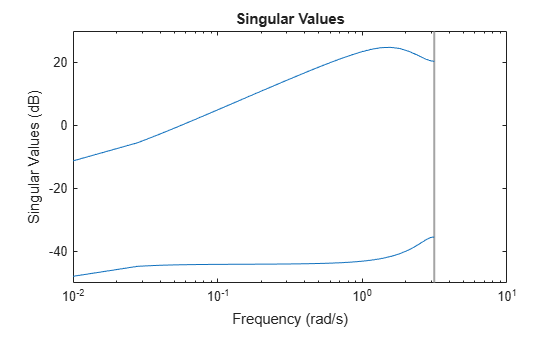Documentation

## Extract Controller

This example shows how to obtain an LTI representation of an unconstrained MPC controller using `ss`. You can use this to analyze the frequency response and performance of the controller.

Define a plant model. For this example, use the CSTR model described in Design Controller Using MPC Designer.

```A = [-0.0285 -0.0014; -0.0371 -0.1476]; B = [-0.0850 0.0238; 0.0802 0.4462]; C = [0 1; 1 0]; D = zeros(2,2); CSTR = ss(A,B,C,D); CSTR.InputGroup.MV = 1; CSTR.InputGroup.UD = 2; CSTR.OutputGroup.MO = 1; CSTR.OutputGroup.UO = 2;```

Create an MPC controller for the defined plant using the same sample time, prediction horizon, and tuning weights described in Design MPC Controller at the Command Line.

`MPCobj = mpc(CSTR,1,15);`
```-->The "ControlHorizon" property of the "mpc" object is empty. Assuming 2. -->The "Weights.ManipulatedVariables" property of "mpc" object is empty. Assuming default 0.00000. -->The "Weights.ManipulatedVariablesRate" property of "mpc" object is empty. Assuming default 0.10000. -->The "Weights.OutputVariables" property of "mpc" object is empty. Assuming default 1.00000. for output(s) y1 and zero weight for output(s) y2 ```
```MPCobj.W.ManipulatedVariablesRate = 0.3; MPCobj.W.OutputVariables = [1 0];```

Extract the LTI state-space representation of the controller.

`MPCss = ss(MPCobj);`
```-->Converting model to discrete time. -->The "Model.Disturbance" property of "mpc" object is empty: Assuming unmeasured input disturbance #2 is integrated white noise. Assuming no disturbance added to measured output channel #1. -->The "Model.Noise" property of the "mpc" object is empty. Assuming white noise on each measured output channel. ```

Convert the original `CSTR` model to discrete form using the same sample time as the MPC controller.

`CSTRd = c2d(CSTR,MPCss.Ts);`

Create an LTI model of the closed-loop system using `feedback`. Use the manipulated variable and measured output for feedback, indicating a positive feedback loop. Using negative feedback would lead to an unstable closed-loop system, because the MPC controller is designed to use positive feedback.

`CLsys = feedback(CSTRd,MPCss,1,1,1);`

You can then analyze the resulting feedback system. For example, verify that all closed-loop poles are within the unit circle.

`poles = eig(CLsys)`
```poles = 6×1 complex 0.5513 + 0.2700i 0.5513 - 0.2700i 0.6131 + 0.1110i 0.6131 - 0.1110i 0.9738 + 0.0000i 0.9359 + 0.0000i ```

You can also view the system frequency response.

`bode(CLsys)`